# AP Physics C: Electricity and Magnetism Practice Test 9

### Test Information11 questions15 minutes

1. Two identical capacitors are hooked in parallel to an external circuit. Which of the following quantities must be the same for both capacitors?

I. the charge stored on the capacitor

II.  the voltage across the capacitor

III. the capacitance of the capacitor

2. A 2 μF capacitor is connected directly to a battery. When the capacitor is fully charged, it stores 600 μC of charge. An experimenter replaces the 2 μF capacitor with three 18 μF capacitors in series connected to the same battery. Once the capacitors are fully charged, what charge is stored on each capacitor?

3. A spherical conductor carries a net charge. How is this charge distributed on the sphere?

4.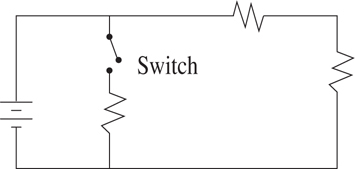Three resistors are connected to a battery as shown in the diagram above. The switch is initially open. When the switch is closed, what happens to the total voltage, current, and resistance in the circuit?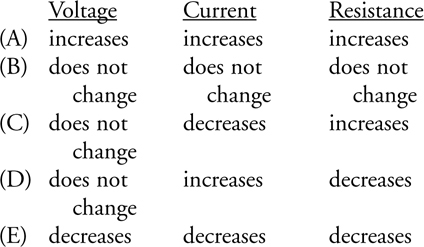Voltage     Current     Resistance

5.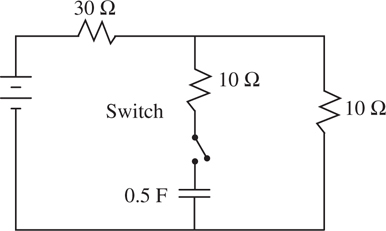In the circuit shown above, the 0.5-F capacitor is initially uncharged. The switch is closed at time t = 0. What is the time constant (the time for the capacitor to charge to 63% of its maximum charge) for the charging of this capacitor?

6.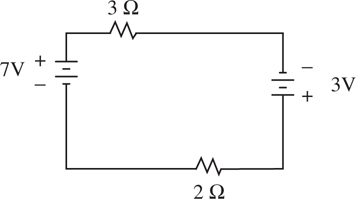In the circuit shown above, what is the current through the 3 Ω resistor?

7. A light bulb rated at 100 W is twice as bright as a bulb rated at 50 W when both are connected in parallel directly to a 100-V source. Now imagine that these bulbs are instead connected in series with each other. Which is brighter, and by how much?

8.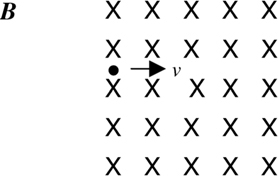A uniform magnetic field B is directed into the page. An electron enters this field with initial velocity v to the right. Which of the following best describes the path of the electron while it is still within the magnetic field?

9.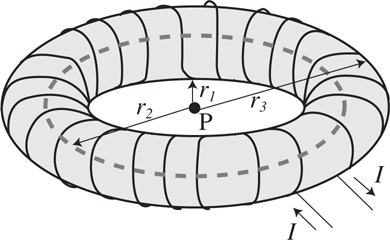Wire is wound around an insulated circular donut, as shown above. A current I flows in the wire in the direction indicated by the arrows. The inner, average, and outer radii of the donut are indicated by r1, r2, and r3, respectively. What is the magnitude and direction of the magnetic field at point P, the center of the donut?

10.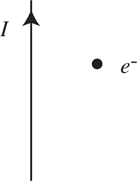A wire carries a current toward the top of the page. An electron is located to the right of the wire, as shown above. In which direction should the electron be moving if it is to experience a magnetic force toward the wire?

11. Which of the following statements about electric and magnetic fields is FALSE: